# 专注，勤学，慎思。戒骄戒躁，谦虚谨慎

just do it

## 一则MySQL派生表优化案例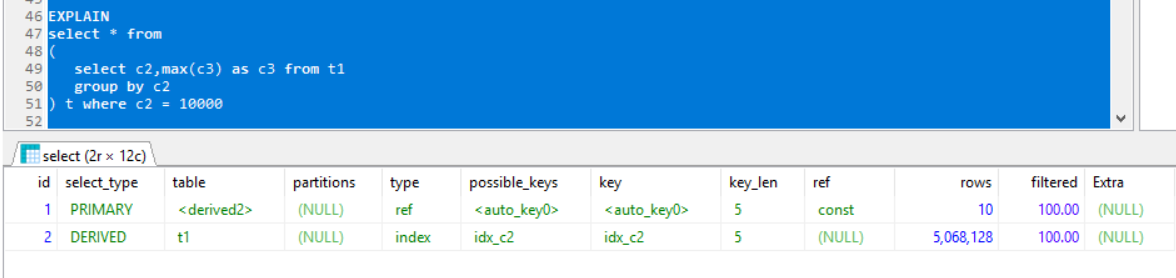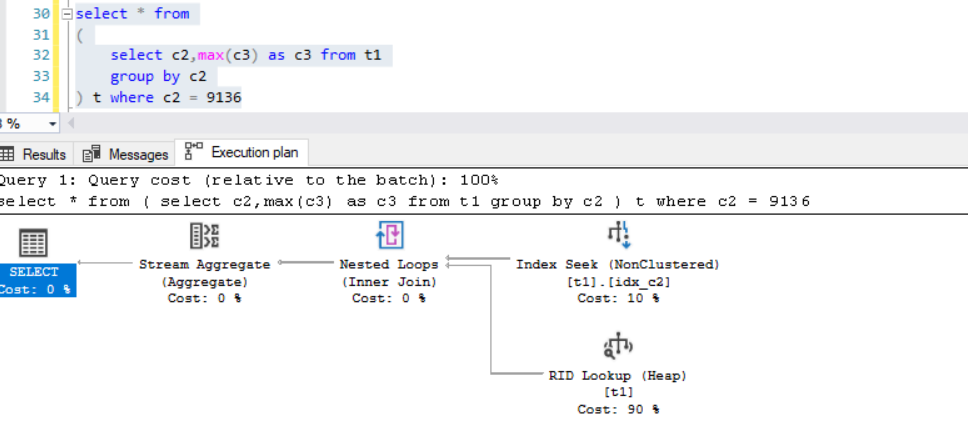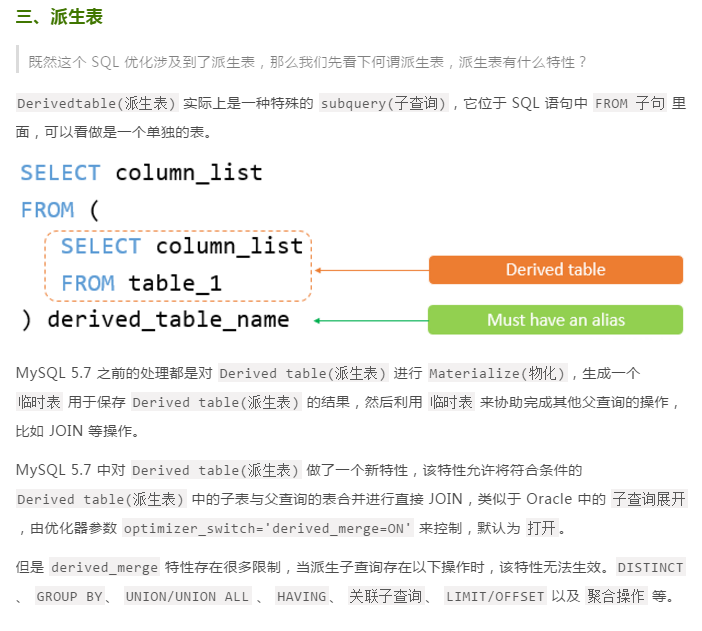CREATE TABLE t1
(
id INT AUTO_INCREMENT PRIMARY key,
c1 INT,
c2 VARCHAR(50),
create_date datetime
);

CREATE TABLE t2
(
id INT  AUTO_INCREMENT PRIMARY key,
c1 INT,
c2 VARCHAR(50),
create_date datetime
);

CREATE INDEX idx_c1 ON t1(c1);
CREATE INDEX idx_c1 ON t2(c1);

CREATE DEFINER=root@% PROCEDURE create_test_data(
IN loop_count INT
)
BEGIN
SET @p_loop = 0;
while @p_loop<loop_count do

SET @p_date = DATE_ADD(NOW(),INTERVAL -RAND()*100 DAY);

INSERT INTO t1 (c1,c2,create_date) VALUES (@p_loop,UUID(),@p_date);

INSERT INTO t2 (c1,c2,create_date) VALUES (@p_loop,UUID(),DATE_ADD(@p_date,INTERVAL RAND()*10000 MINUTE));
INSERT INTO t2 (c1,c2,create_date) VALUES (@p_loop,UUID(),DATE_ADD(@p_date,INTERVAL RAND()*10000 MINUTE));
INSERT INTO t2 (c1,c2,create_date) VALUES (@p_loop,UUID(),DATE_ADD(@p_date,INTERVAL RAND()*10000 MINUTE));
INSERT INTO t2 (c1,c2,create_date) VALUES (@p_loop,UUID(),DATE_ADD(@p_date,INTERVAL RAND()*10000 MINUTE));
INSERT INTO t2 (c1,c2,create_date) VALUES (@p_loop,UUID(),DATE_ADD(@p_date,INTERVAL RAND()*10000 MINUTE));
INSERT INTO t2 (c1,c2,create_date) VALUES (@p_loop,UUID(),DATE_ADD(@p_date,INTERVAL RAND()*10000 MINUTE));
INSERT INTO t2 (c1,c2,create_date) VALUES (@p_loop,UUID(),DATE_ADD(@p_date,INTERVAL RAND()*10000 MINUTE));
INSERT INTO t2 (c1,c2,create_date) VALUES (@p_loop,UUID(),DATE_ADD(@p_date,INTERVAL RAND()*10000 MINUTE));
INSERT INTO t2 (c1,c2,create_date) VALUES (@p_loop,UUID(),DATE_ADD(@p_date,INTERVAL RAND()*10000 MINUTE));
INSERT INTO t2 (c1,c2,create_date) VALUES (@p_loop,UUID(),DATE_ADD(@p_date,INTERVAL RAND()*10000 MINUTE));

SET @p_loop = @p_loop+1;

END while;
END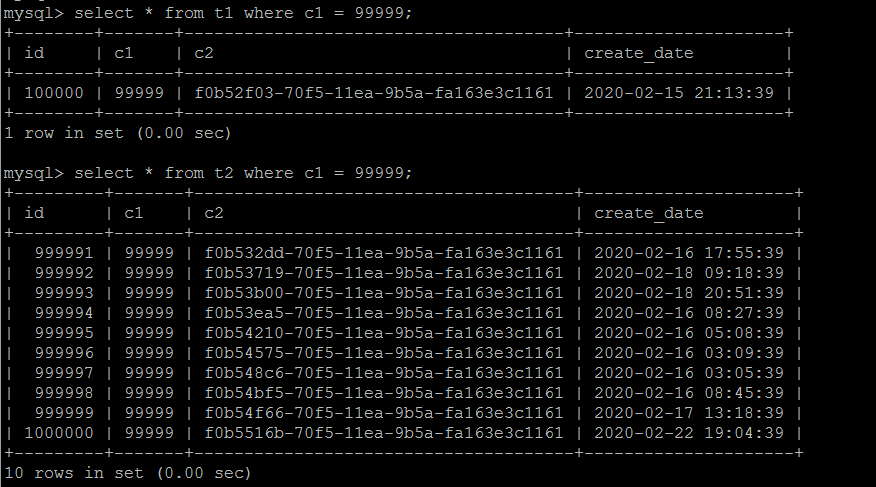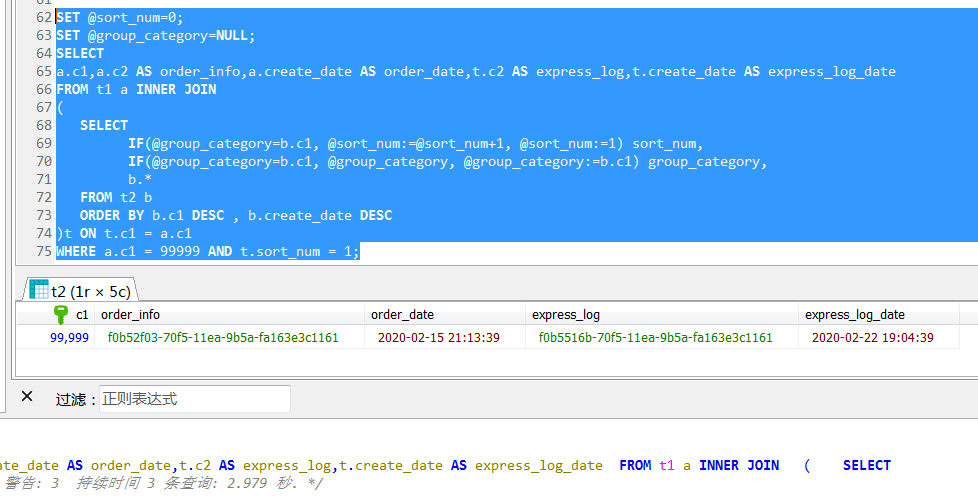1，如果按照预期的方式执行，但是性能并没有达到预期，需要反思是什么因素造成的？
2，如果没有按照预期的方式执行，同样需要反思了，为什么没有按照预期的方式执行，可能会是什么原因造成的？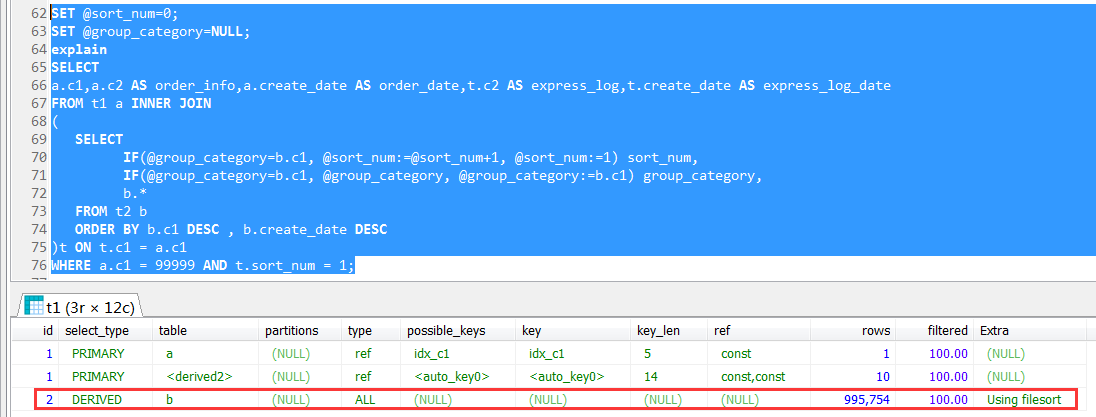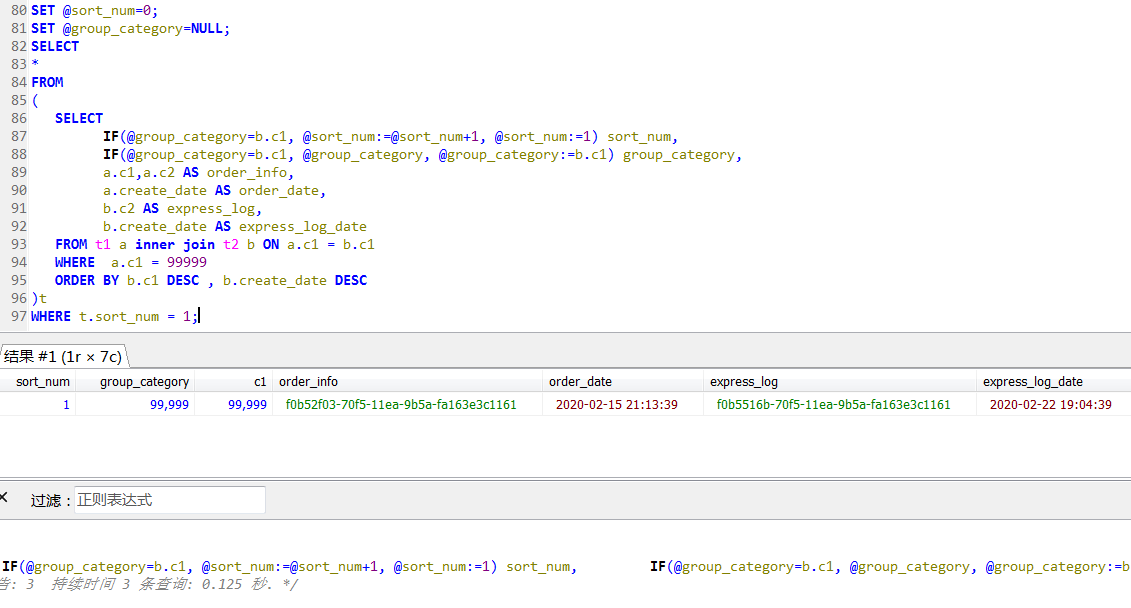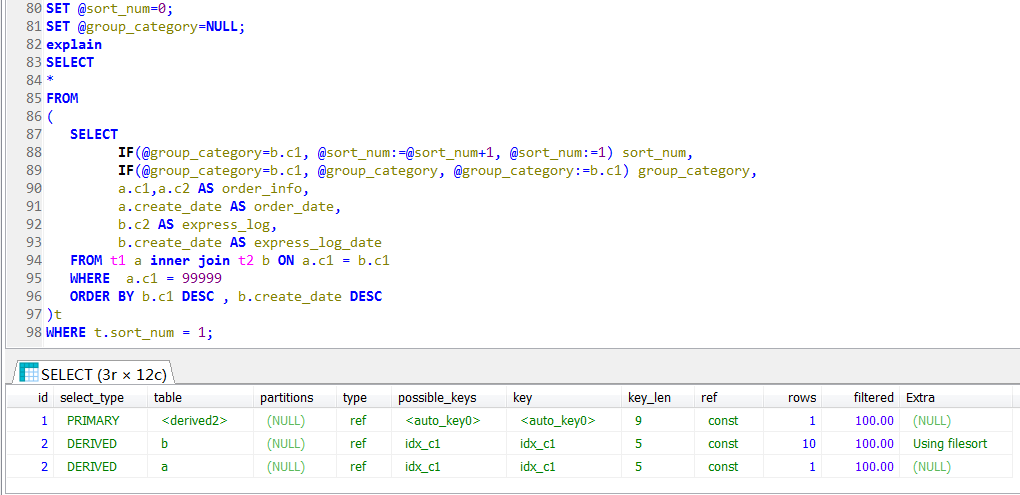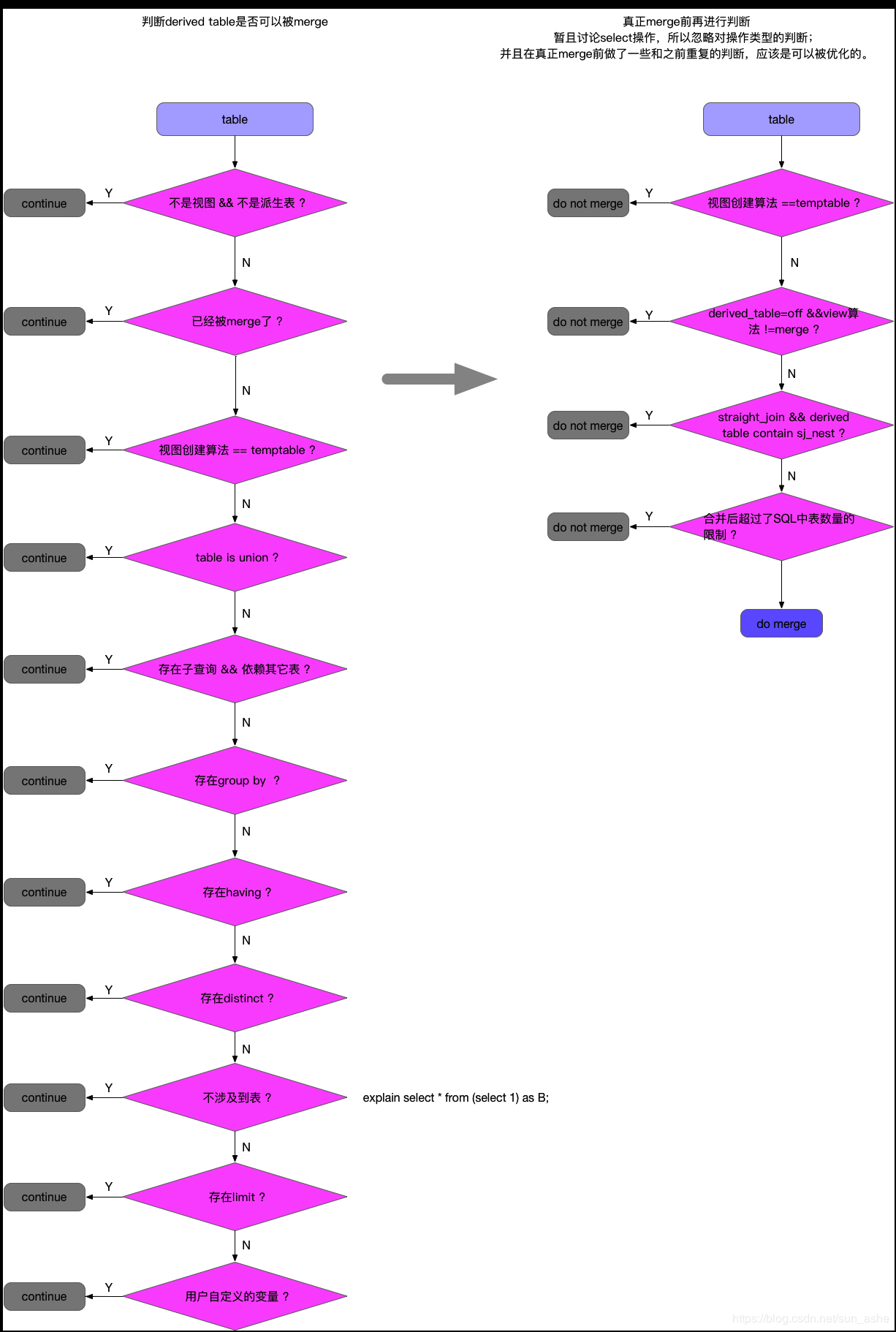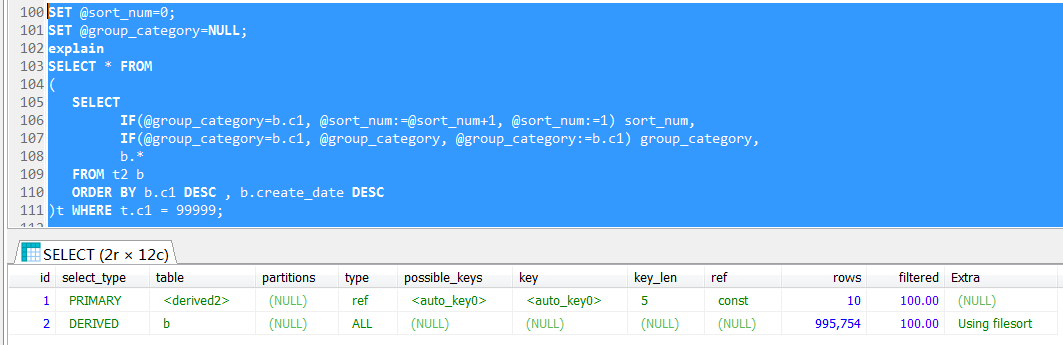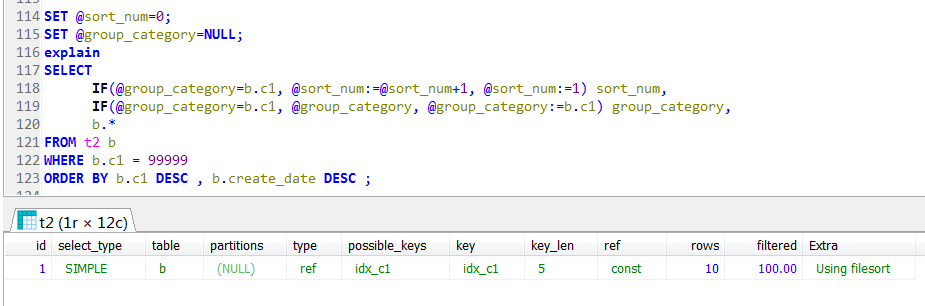MySQL 8.0的分析函数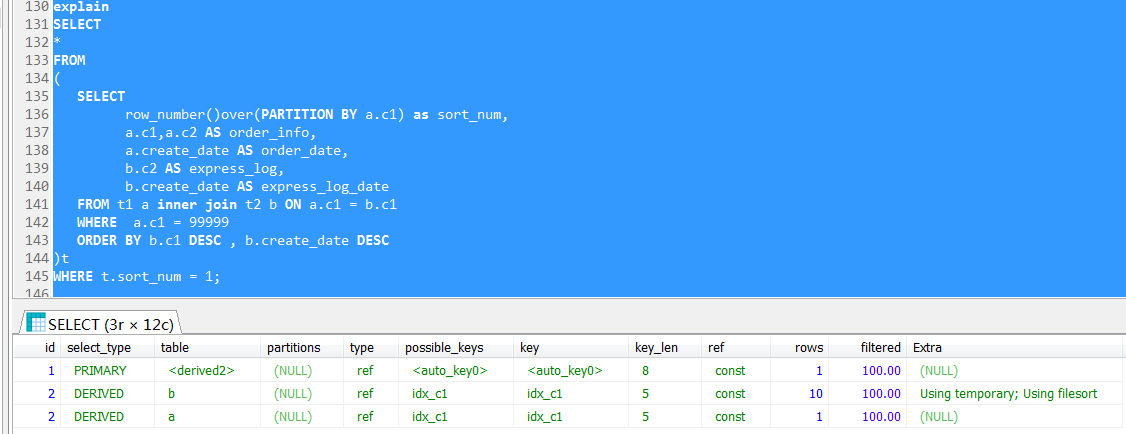demo的sql

SET @sort_num=0;
SET @group_category=NULL;
SELECT
a.c1,a.c2 AS order_info,a.create_date AS order_date,t.c2 AS express_log,t.create_date AS express_log_date
FROM t1 a INNER JOIN
(
SELECT
IF(@group_category=b.c1, @sort_num:=@sort_num+1, @sort_num:=1) sort_num,
IF(@group_category=b.c1, @group_category, @group_category:=b.c1) group_category,
b.*
FROM t2 b
ORDER BY b.c1 DESC , b.create_date DESC
)t ON t.c1 = a.c1
WHERE a.c1 = 99999 AND t.sort_num = 1;

SET @sort_num=0;
SET @group_category=NULL;
SELECT
*
FROM
(
SELECT
IF(@group_category=b.c1, @sort_num:=@sort_num+1, @sort_num:=1) sort_num,
IF(@group_category=b.c1, @group_category, @group_category:=b.c1) group_category,
a.c1,a.c2 AS order_info,
a.create_date AS order_date,
b.c2 AS express_log,
b.create_date AS express_log_date
FROM t1 a inner join t2 b ON a.c1 = b.c1
WHERE  a.c1 = 99999
ORDER BY b.c1 DESC , b.create_date DESC
)t
WHERE t.sort_num = 1;

SELECT
*
FROM
(
SELECT
row_number()over(PARTITION BY a.c1 ORDER BY b.create_date desc) as sort_num,
a.c1,
a.c2 AS order_info,
a.create_date AS order_date,
b.c2 AS express_log,
b.create_date AS express_log_date
FROM t1 a inner join t2 b ON a.c1 = b.c1
WHERE b.c1 = 99999
)t
WHERE t.sort_num = 1;

posted on 2020-03-29 18:40  MSSQL123  阅读(1550)  评论(0编辑  收藏  举报